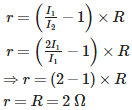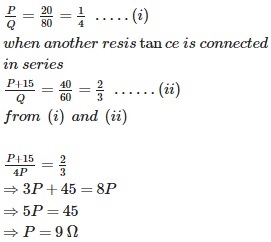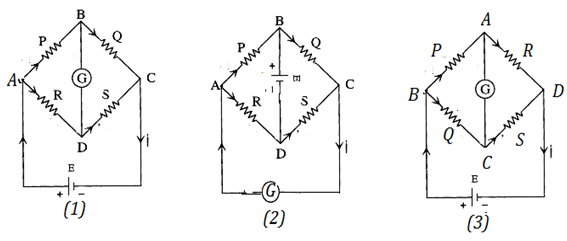# Current Electricity - Online Test

Q1. Two cells of 1.25 V and 0.75 V are connected in series with anode of one connected to cathode of the other . The effective voltage will be
Explaination / Solution:

The cells support each other.
Q2. Two cells of 1.25 V and 0.75 V are connected in series with anode of one connected to anode of the other . The effective voltage will be
Explaination / Solution:

The cells are in opposition.
Q3. The instrument for the accurate measurement of the e.m.f of a cell is
Explaination / Solution:

Both potentiometer and voltmeter are devices to measure potential difference. E M F is the terminal p.d between the electrodes of a cell in open circuit, i.e., when no current is drawn from it. Potentiometer measures the potential difference using null deflection method, where no current is drawn from the cell; whereas voltmeter needs a small current to show deflection. So, accurate measurement of p.d is done using a potentiometer.

Q4. Potentiometer measures the potential difference more accurately than a voltmeter, because
Explaination / Solution:

Potentiometer measures the potential difference using null deflection method, where no current is drawn from the cell; whereas voltmeter needs a small current to show deflection. So, accurate measurement of p.d is done using a potentiometer.

Q5. In a potentiometer experiment, for measuring internal resistance of a cell, the balance point has been obtained on the fourth wire. The balance point can be shifted to fifth wire by
Explaination / Solution:

If the current due to the auxillary battery is decreased, the potential gradient will be decreases, so the balancing length increases. Thus null point will move to fifth wire.

Q6. It is observed in a potentiometer experiment that no current passes through the galvanometer, when the terminals of the cell are connected across a certain length of the potentiometer wire. On shunting the cell by a 2 Ω resistance, the balancing length is reduced to half. The internal resistance of the cell is
Explaination / Solution:

The internal resistance of the cell is given by:Q7. The sensitivity of the potentiometer can be increased by:
Explaination / Solution:

A potentiometer is considered to be sensitive if the potential gradient is low. Such a potentiometer can measure very small changes in potential difference. Increasing the length of the potentiometer wire decreases the potential gradient. Its sensitivity increases. Increasing potential gradient decreases the sensitivity. increasing the emf of the primary cell and by decreasing the length, potential gradient increases.

Q8. Two resistances are connected in the two gaps of a meter bridge. The balance points is 20 cm from the zero end. When a resistance of 15 ohm is connected in series with the smaller of the two resistances, the null point shifts to 40 cm. The smaller of the two resistances has the value (in ohm)
Explaination / Solution:

Let the resistances be P and Q. When balanced,Q9. In a meter bridge, when galvanometer & cell positions are interchanged
Explaination / Solution:

The battery in the circuit(1) shown is connected between A and C, while the galvanometer is connected between B and D. In the bridge balanced condition,When the battery and the galvanometer are interchanged, the circuit takes the form (2). The circuit (2) can be re drawn as circuit (3). The ratio of the resistances in the bridge balanced condition is which is same as,
Q10. An ammeter together with an unknown resistance in series is connected across two identical batteries each of emf 1.5 V. When the batteries are connected in series, the galvanometer records a current of 1A and when the batteries are in parallel, the current is 0.6A. Then the internal resistance of the battery is
Explaination / Solution:

When the batteries are in series, the internal resistances are in series. Both e m f and the internal resistances add up.

from equation (i) and (ii)